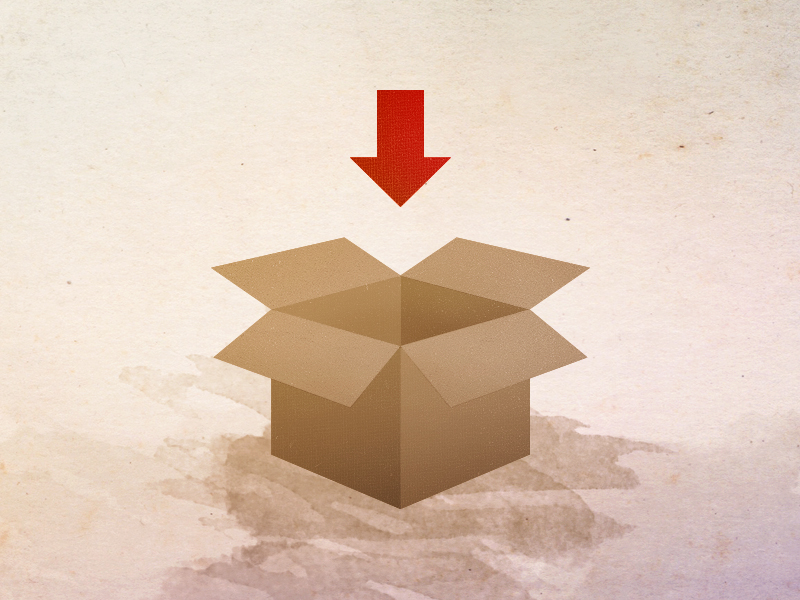# JavaScript #2 ~ Values & Variables

hjbaek91·2021년 5월 18일
0

목록 보기
3/20

## Values

Values are pieces of data that are stored and used for certain programming purposes. In programming terms, a value is the smallest unit of information in a code.

## Variables

One of the most useful functions of a value is that it can be stored into variables. This way, it can be re-used anytime whenever needed. As such, whenever we need to find a certain value, all we would need to do is look for the right variable that stores the value.

let firstName = 'John';
let age = 8;For an easier approach, we can think of storing things into a box. In this case, the box that is able to store things would be the variable and the things that would go into the box would be the values. Whenever we need to look for the values we want, we would simply find the name of the box.

But how would we be able to see the results? We would simply use the console.log method to visually make it appear within the chrome developer tool.

let firstName = 'John';
console.log(firstName); // John

Another useful function of variables is that we can change the value inside the variable. Say that we have a variable of 'firstName' with a value of 'John' inside and a bunch of console.log lines. If we decide to change the value inside the variable, what would be the appropriate approach?

let firstName = 'John';
console.log(firstName); // John
console.log(firstName); // John
console.log(firstName); // John

Instead of trying to literally change each individual line of code one-by-one, we could simply just change the value itself. This way, all the other lines would change automatically.

let firstName = 'David';
console.log(firstName); // David
console.log(firstName); // David
console.log(firstName); // David

Although simple, revisiting these topics again reinforces very crucial basic knowledge that helps me become a better developer in the future. As such I will continue to strive through this journey 🙂